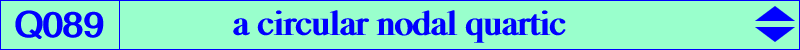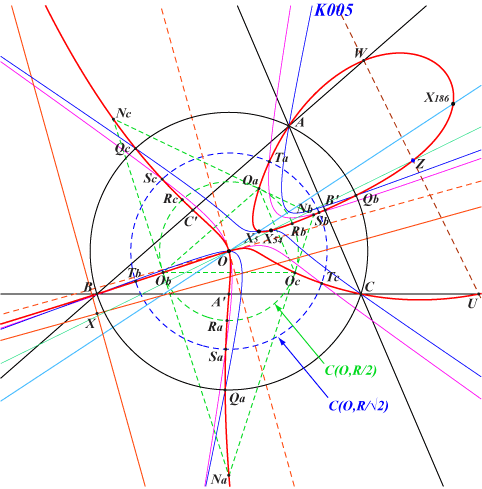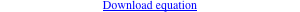too complicated to be written here. Click on the link to download a text file.X(3), X(5), X(54), X(186), X(2574), X(2575), X(6150) Z = X(6150) = midpoint of X(3)X(1157) Qa, Qb, Qc points on (O) and K005 A', B', C' midpoints of ABC U, V, W, Z on the trilinear polar of X(1994) i.e. the orthotransversal of X(5), Z also on X(3)X(54) Oa, Ob, Oc midpoints of OA, OB, OC other points described belowQ089 is the locus of the centers of central focal circum-cubics passing through O. See the related curves K026 and Q019 where O is replaced by H and G. For instance, when X = X(5) we obtain K465. Q089 is a circular nodal quartic with node O, the nodal tangents are parallel to the asymptotes of the Jerabek hyperbola. The real asymptotes of Q089 are also parallel to these same lines and concur at the midpoint X = X(14156) of X(3)X(1568). The imaginary tangents concur at X(186), the singular focus of Q089. Q089 is inversible in the circle with center O, radius R/√2. Thus, Q089 meets this circle at six points Sa, Sb, Sc, Ta, Tb, Tc where the tangents pass through O. The inversive images of the midpoints A', B', C' are Na, Nb, Nc which are also the midpoints of O and the vertices of the tangential triangle. Q089 meets C(O, R/2) at Oa, Ob, Oc and three other points Ra, Rb, Rc which are the inversive images of Qa, Qb, Qc.